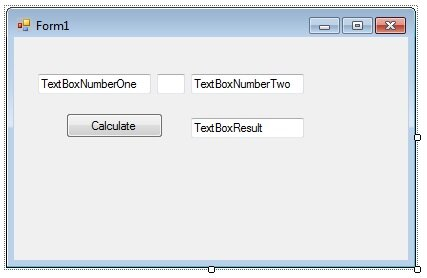# Visual Basic Arithmetic Operators

“Arithmetic Operators” is a rather obtuse name for, what are, very simple maths functions such as addition, subtraction, multiplication and division.   Have I mentioned that computer programmers love to give a simple concept as intimidating a name as possible?  Well they do, time and time again.  Perhaps its a means to blind you with science, I’m not sure.  I’m sure they’d blame the mathematicians who invented the term but come on guys, you had the chance to make this far more accessible to the layman.  I ought to be thankful of course, I wouldn’t be here if they’d made programming simple, but still…

As you’d expect, it is extremely easy to implement these Arithmetic Operators in Visual Basic.  The syntax for the operators are:

• - for subtraction
• / for division
• * for multiplication

Create a new Project called Calculator

• Extend the Form so it’s slightly wider than by default
• Add 4 textboxes called TextBoxNumberOne, TextBoxOperator, TextBoxNumberTwo and TextBoxResult.
• Add a button to the Form balled ButtonCalculate with the Text “Calculate”.
• Style it so it looks as follows:In the ButtonCalculate Click Event add the following code

```Dim numberOne As Integer = CInt(TextBoxNumberOne.Text)
Dim numberTwo As Integer = CInt(TextBoxNumberTwo.Text)

If TextBoxOperator.Text = "+" Then
TextBoxResult.Text = numberOne + numberTwo
ElseIf TextBoxOperator.Text = "-" Then
TextBoxResult.Text = numberOne - numberTwo
ElseIf TextBoxOperator.Text = "*" Then
TextBoxResult.Text = numberOne * numberTwo
Else
TextBoxResult.Text = numberOne / numberTwo
End If
```

The first two lines are declaring the variables and assigning values on declaration.  The values are the Integer values of TextBoxNumberOne and Two.

The next block is an If/ElseIf/Else block which looks at the contents of TextBoxOperator, and depending on the contents, execute that operator and put the result in TextBoxResult.  For example, if there is a + in the textbox, add the two numbers and show the result.

Run the program and play with it.  You’ve created a simple calculator in 12 lines of code!

See if you can break it.  We’ve made some pretty fundamental assumptions in this routine.  See if you can spot them and break them.  Some people are naturally excellent at doing this and often I recommend they look at becoming testers, after they’ve learnt to code of course.

Let me know how you broke this routine.